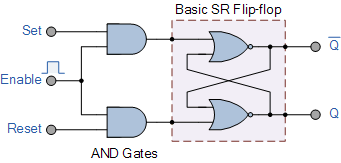# Conversion of Flip-flops

## Conversion of Flip-flops

We have seen throughout this Electronics Tutorial section on Sequential Logic that a flip-flop will remain in one of its two stable states indefinitely until some form of external trigger pulse is applied to make it change state.As flip-flops are bistable devices, these sequential circuits are sometimes called “latches” because their outputs are locked or latched onto their input state until there is another change to its input condition.

We have also seen that the bistable flip-flop is the most basic storage element in a sequential logic circuit and can be configured to produce simple memory elements by interconnecting two inverting gates to generate feedback. Note that a combinational logic circuit does not require any form of memory and therefore does not use flip-flops. However, sequential logic circuits do have memory and therefore use various types of flip-flop designs to remember their present states.

The interconnection of digital logic gates to produce a memory device leads to applications such as switch debounce circuits, shift registers and counters, etc. Also, memory elements made from bistable latches form the basis of accumulators and registers on which a computer, or micro-controller, does its complex arithmetic.The most basic way to create a single one-bit flip-flop is to use two NOR gates gates as shown. By using cross-coupled gates and feeding the output from one gate to the input of the other, (inputs-outputs interchanged) the circuit has a closed-loop (positive feedback) so its output depends on the state of the inputs, making the circuit sequential and having memory.

Flip-flops also belong to a category of digital switching circuits called Multivibrators. The basic bistable multivibrator is a type of regenerative circuit that has two active digital gates designed so that when one digital gate conducts, the other gate is cut-off and vice versa. These two digital gates produce two stable outputs both HIGH and LOW in which one is the complement of the other.

But we can make many different types of flip-flop circuits both asynchronous and synchronous (an asynchronous flip-flop does not require a clock signal input, but a synchronous one does) from either basic cross-coupled NAND Gates and NOR Gates with the conversion of flip-flops between the different types sometimes a bit confusion.

There are basically four different types of flip flops and these are:

• 1. Set-Reset (SR) flip-flop or Latch
• 2. JK flip-flop
• 3. D (Data or Delay) flip-flop
• 4. T (Toggle) flip-flop

So to help us understand better the different types of flip-flops available, the following sequential logic tutorial shows us how we can make the conversion of flip-flops from one type to another simply by modifying the inputs of a particular type of a flip-flop starting with the SR flip-flop.

## The Set-Reset SR Flip-flop

The most basic of all the bistable latches and bistable multivibrators is the set-rest (SR) flip-flop. The basic SR flip-flop is an important bistable circuit because all the other types of flip-flop are built from it. The SR flip-flop is constructed using two cross-coupled digital NAND gates such as the TTL 74LS00, or two cross-coupled digital NOR gates such as the TTL 74LS02.

Generally SR bistables and flip-flops are said to be transparent because their outputs change or respond immediately to changes in their inputs. Also since they consist of digital logic gates along with feedback, SR flip-flops are regarded as asynchronous sequential logic circuits.

The basic SR flip-flop has two inputs S (set) and R (reset) and two outputs Q and Q with one of these outputs being the complement of the other. Then the SR flip-flop is a two-input, two-output device. Consider the circuits below.

### Basic NAND and NOR SR Flip-flops